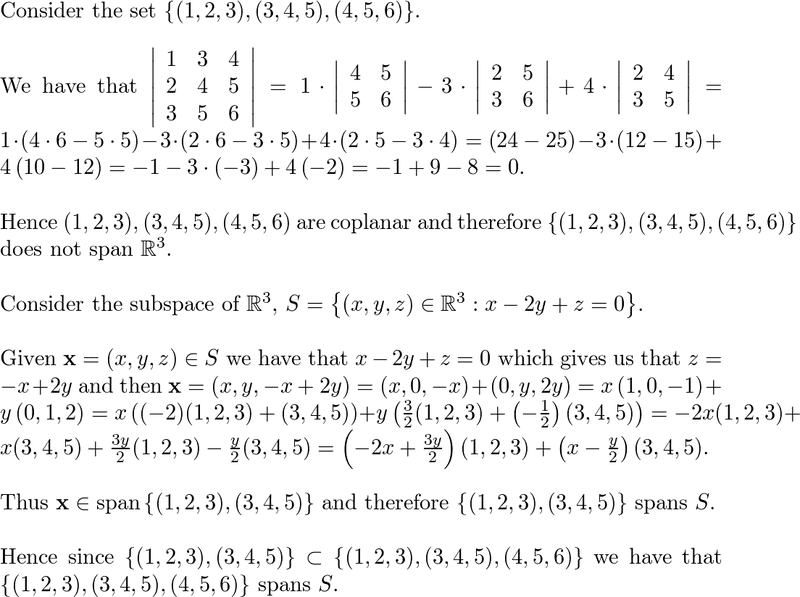# Show that a set of vectors spans a subspace

• mattqchou

## Homework Statement

Show that {(1, 2, 3), (3, 4, 5), (4, 5, 6)} does not span R3. Show that it spans the subspace of R3 consisting of all vectors lying in the plane with the equation x - 2y + z = 0.

## The Attempt at a Solution

A = [ 1 3 4 ; 2 4 5; 3 5 6] and reduced it to row-echelon form to determine rank. I found that rank(A) = 2. Therefore, it can't span R3, since rank(A) < n for Rn.

But then I need to show that it spans the plane given by the equation x - 2y + z = 0.

I thought that perhaps rewriting the problem as: z = -x + 2y and plugging back in for z. But that doesn't really tell me anything.

I also tried to show the span by demonstrating where it has linear combinations by writing span(F) = span{ax - 2by + cz = 0} but I don't know what to do from here.

I was able to find the solution in the solutions manual, but I can't decipher what it's saying or how it did it. It's not using a method described in the book.Could someone explain this better or give me another method to figure out whether a set spans a subspace?

#### Attachments

They find explicit coefficients for the vectors (1,2,3) and (3,4,5) to express every vector in the plane as linear combination of these vectors.
The coefficients are (-2x+3y/2) and (x-y/2). Multiply them with the vectors, simplify and you get a general vector in the discussed plane.

They find explicit coefficients for the vectors (1,2,3) and (3,4,5) to express every vector in the plane as linear combination of these vectors.
The coefficients are (-2x+3y/2) and (x-y/2). Multiply them with the vectors, simplify and you get a general vector in the discussed plane.
Hi,

Could you explain how you find explicit coefficients? I've never heard of this method in my class before. I also can't find that in the book or the glossary.

Thanks!

See the calculation above, it does exactly this.
It starts with an arbitrary vector in the plane and assumes that it can be written as ##\alpha (1,2,3) + \beta (3,4,5)## and then solves for the two unknowns.

But then I need to show that it spans the plane given by the equation x - 2y + z = 0.

I thought that perhaps rewriting the problem as: z = -x + 2y and plugging back in for z. But that doesn't really tell me anything.
Sure it does. You now have three equations that describe the vectors that lie in that plane:
\begin{align*}
x &= s \\
y &= t \\
z &= -s + 2t
\end{align*} or in vector form,
$$\begin{pmatrix} x \\ y \\ z \end{pmatrix} = s\begin{pmatrix} 1 \\ 0 \\ -1\end{pmatrix} + t\begin{pmatrix} 0 \\ 1 \\ 2 \end{pmatrix}.$$ Can you take it from there?